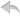注册    登录创作新主题

Python
 python开源   Django   Python   DjangoApp   pycharm
DATA
 docker   Elasticsearch

 问与答   闲聊   招聘   翻译   创业   分享发现   分享创造   求职   区块链   支付之战
WEB开发
 linux   MongoDB   Redis   DATABASE   NGINX   其他Web框架   web工具   zookeeper   tornado   NoSql   Bootstrap   js   peewee   Git   bottle   IE   MQ   Jquery

Python88.com
 反馈   公告   社区推广

Python社区  »  MongoDB

# mongodb中的条件更新多?[副本]

Patrickkx • 2 年前 • 612 次点击

``````db.usercollection.find(
{\$where: "(this.name.length > 40)"}
).limit(2);

output :error: {
"\$err" : "TypeError: Cannot read property 'length' of undefined near '40)' ",
"code" : 16722
}
``````

Python社区是高质量的Python/Django开发社区•   1 楼 Anban    2 年前 此查询将给出字段值和长度: ``````db.usercollection.aggregate([ { \$project: { "name": 1, "length": { \$strLenCP: "\$name" } }} ]) ``````•   2 楼 Fumiya Karasawa    3 年前 查询 ``` \$where ``` 和 ``` \$expr ``` ``` \$regex ``` 比 ``` ``` , ``` \$出口 ``` . ``````db.usercollection.find({ "name": /^[\s\S]{40,}\$/, // name.length >= 40 }) or db.usercollection.find({ "name": { "\$regex": "^[\s\S]{40,}\$" }, // name.length >= 40 }) `````` 此查询的含义与 ``````db.usercollection.find({ "\$where": "this.name && this.name.length >= 40", }) or db.usercollection.find({ "name": { "\$exists": true }, "\$expr": { "\$gte": [ { "\$strLenCP": "\$name" }, 40 ] } }) `````` ``````# find \$where: 10529.359ms \$expr: 5305.801ms \$regex: 2516.124ms # count \$where: 10872.006ms \$expr: 2630.155ms \$regex: 158.066ms ``````•   3 楼 Udara Gunathilake    4 年前 我有一个类似的场景,但在我的例子中字符串不是一级属性。它在一个物体里面。在这里我找不到合适的答案。所以我想和大家分享我的解决方案(希望这能帮助任何有类似问题的人)。 ``````Parent Collection { "Child": { "name":"Random Name", "Age:"09" } } `````` `````` db.getCollection('Parent').find({\$where: function() { for (var field in this.Child.name) { if (this.Child.name.length > 10) return true; } }}) ``````•   4 楼 Rajdeep Gautam    3 年前 ``````db.usercollection.find({ \$where: 'this.name.length < 4' }) ``````•   5 楼 chridam    4 年前 对于MongoDB 3.6及更新版本: 这个 ``` \$expr ``` ``` \$strLenCP ``` 运算符检查字符串的长度,如下所示: ``````db.usercollection.find({ "name": { "\$exists": true }, "\$expr": { "\$gt": [ { "\$strLenCP": "\$name" }, 40 ] } }) `````` 对于MongoDB 3.4及更新版本: ``` \$redact ``` 管道运算符,允许您使用 ``` \$cond ``` 操作员和使用特殊操作 ``` \$\$KEEP ``` 在逻辑条件为真或 ``` \$\$PRUNE ``` 删除条件为假的文档。 这个操作类似于 ``` \$project ``` 选择集合中的字段并创建新字段的管道,该字段保存逻辑条件查询的结果,然后 ``` \$match ``` ``` \$修订 ``` 使用单管道级,效率更高。 至于逻辑条件,有 String Aggregation Operators ``` \$strLenCP公司 ``` 运算符检查字符串的长度。如果长度是 ``` \$gt ``` 一个指定的值,则这是一个真正的匹配,并且文档被“保留”。否则将被“修剪”并丢弃。 ``````db.usercollection.aggregate([ { "\$match": { "name": { "\$exists": true } } }, { "\$redact": { "\$cond": [ { "\$gt": [ { "\$strLenCP": "\$name" }, 40] }, "\$\$KEEP", "\$\$PRUNE" ] } }, { "\$limit": 2 } ]) `````` 如果使用 ``` \$where ``` ,请尝试不带括号的查询: ``````db.usercollection.find({\$where: "this.name.length > 40"}).limit(2); `````` ``````db.usercollection.find({name: {\$type: 2}, \$where: "this.name.length > 40"}).limit(2); `````` 或: ``````db.usercollection.find({name: {\$exists: true}, \$where: "this.name.length > 40"}).limit(2); `````` MongoDB计算非- ``` \$哪里 ``` 之前的查询操作 ``` ``` 表达式与非- ``` \$哪里 ``` 查询语句可以使用索引。更好的性能是将字符串的长度存储为另一个字段,然后可以对其进行索引或搜索;应用 ``` \$哪里 ``` ``` \$哪里 ``` 当您无法以任何其他方式构造数据时,或者当您正在处理 一种避免使用 ``` ``` 接线员是 ``` \$regex ``` 接线员。考虑以下搜索 ``````db.usercollection.find({"name": {"\$type": 2, "\$regex": /^.{41,}\$/}}).limit(2); `````` 注意 docs : 针对索引中的值的表达式,该值可以比 收集扫描。如果 表达式是前缀表达式,这意味着 范围从该前缀开始,并且仅与 在该范围内的索引。 如果正则表达式以 插入符号 ``` (^) ``` 或左锚 ``` (\A) ``` ,后跟一个简单的字符串 符号。例如,regex ``` /^abc.*/ ``` 将通过 仅与以开头的索引中的值匹配 ``` abc ``` 另外,当 ``` /^a/, /^a.*/, ``` 和 ``` /^a.*\$/ ``` 字符串有不同的性能特征。所有这些 如果存在适当的索引,表达式将使用索引;但是, ``` /^a.*/ ``` ,和 ``` ``` 比较慢。 ``` /^a/ ``` 扫描后可以停止 匹配前缀。